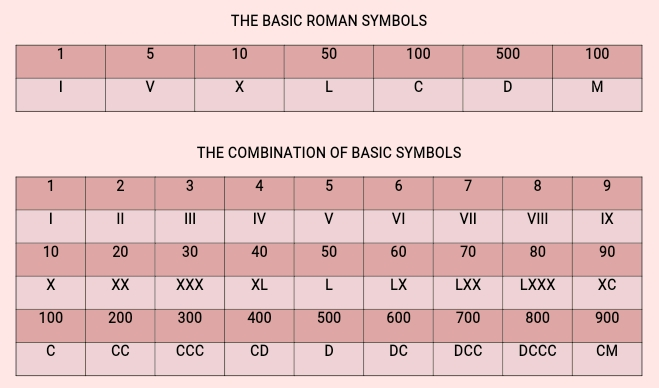# Roman Numerals (I – C)

Last Updated on September 25, 2018 by Alabi M. S.

MATHEMATICS

FIRST TERM

FOURTH WEEK

BASIC 4

THEME:

TOPIC: Roman Numerals (I – C)

PERFORMANCE OBJECTIVES

• By the end of the lesson, the pupils should have attained the following objectives (cognitive, affective and psychomotor) and be able to:
1. count Roman numerals up to 100 (I to C);
2. solve problems on quantitative reasoning use of roman numerals.

ENTRY BEHAVIOR

The pupils are required to already have learned to counting up to 1,000.

INSTRUCTIONAL MATERIALS

• The teacher will teach the lesson with the aid of:
1. Roman numeral charts
2. Flash cards
3. Charts
4. Flash cards
5. Number charts.

METHOD OF TEACHING

1. Explanatory
2. Discussion
3. Demonstration

REFERENCE MATERIALS

1. Scheme of Work
2. 9 – Years Basic Education Curriculum
3. New Method Mathematics Book 6
4. MathisFun
5. All Relevant Material
6. Online Information

CONTENT OF THE LESSON

ROMAN NUMERALS (I – C)

Basic Application

Examples

1 = I

2 = II

3 = III

4 = 5 – 1 = V – I = IV,

Note

Rule number 1,

• When a larger symbol comes after the smaller symbol, it is substation. That is, IV = V – I = 4

5 = V

6 = V + I = VI

Rule number 2,

• When a smaller symbol comes after the larger symbol, it is addition. That is, V + I = 6.

Rule number 3,

• Don’t use the same symbol more than three times in a row.Exercise 1

Read Roman numerals from 11 – 19.

Exercise 2

Write Roman numerals from 21 – 30.

PRESENTATION

• To deliver the lesson, the teacher adopts the following steps:
1. To introduce the lesson, the teacher revises the previous lesson. Based on this, he/she asks the pupils some questions;
2. Guides pupils to use fingers to represent numbers in Roman numerals I – X (1 to 10);
3. Guides pupils to form Roman numeral – I (one finger), V (five fingers), X (ten fingers);
4. Pupil’s Activities – Read and write Roman numerals up to 10.;
5. Uses Roman numeral chart to lead pupils to read and write the Roman numerals I to C;
6. Pupil’s Activities – Study the Roman numerical chart and flash cards.
7. Pupil’s Activities – Practice the number formation system for Roman numerical ( I × C) using fingers.
8. Pupil’s Activities – Write all the Roman numerals I to C in their exercise book.
9. Gives examples on quantitative aptitude, e.g. find the missing number below;
10. Pupil’s Activities – Solve examples on quantitative aptitude involving roman numerals.

CONCLUSION

• To conclude the lesson for the week, the teacher revises the entire lesson and links it to the following week’s lesson.

LESSON EVALUATION

Pupils to:

1. read given Roman numerals and state the corresponding values in the Hindu/Arabic Form.
2. write the value of the Roman numeral I – C in the Hindu/Arabic form;
3. write 1 – 100 in roman numerals;
4. solve problems on quantitative aptitude.